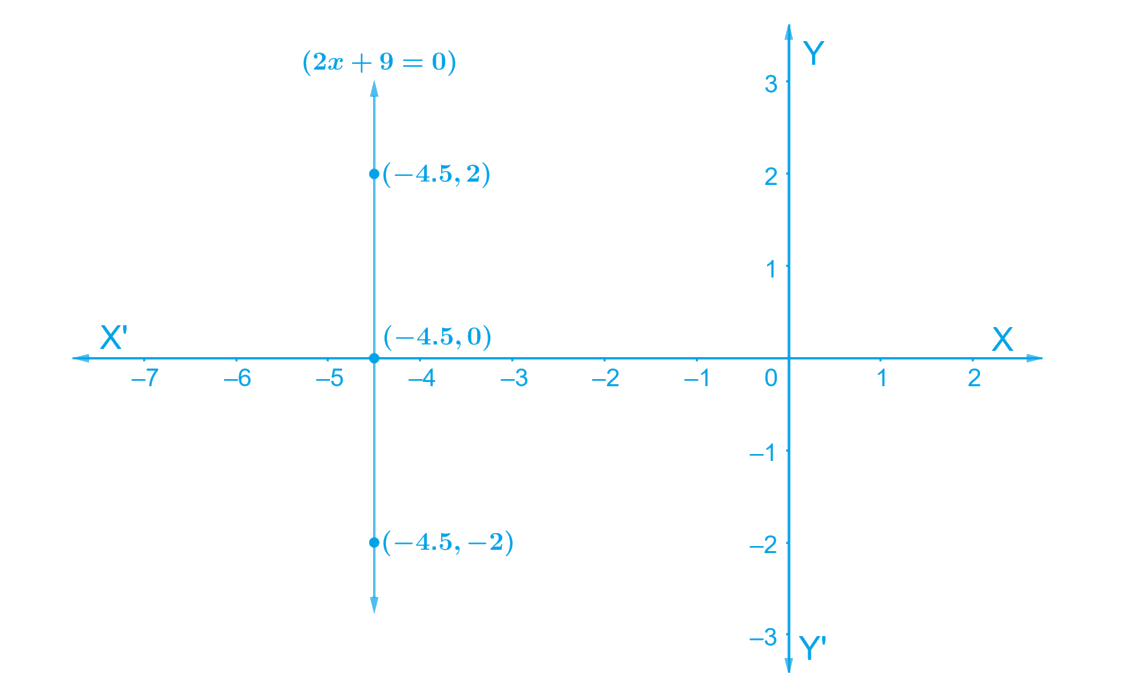# Ex.4.4 Q2 Linear Equations in Two Variables Solution - NCERT Maths Class 9

Go back to  'Ex.4.4'

## Question

Give the geometric representations of

$$2x + 9 = 0$$ as an equation

(i) in one variable

(ii) in two variables

Video Solution
Linear Equations In Two Variables
Ex 4.4 | Question 2

## Text Solution

#### Steps:

(i) Given: $$2x + 9 = 0$$ is the Linear Equation -------- Equation (1)

\begin{align} 2 x + 9 &= 0 \\ 2 x &= - 9 \\ x &= \frac { - 9 } { 2 } \\ &= -4.5 \end{align}

Hence, in one variable $$2x + 9 = 0$$ represents a point as shown in the following figure.(ii) Given: $$2x + 9 = 0$$ is the Linear Equation ------- Equation (1)

We know that $$2x + 9 = 0$$ can be written as $$2x + 0y + 9 = 0$$ as a linear equation in variables $$x$$ and $$y$$.

Value of $$y$$ is always $$0$$. However, $$x$$ must satisfy the relation $$2x + 9= 0$$

\begin{align} \text { i.e. } \;\;\; x &= \frac { - 9 } { 2 } \\ & = - 4.5 \end{align}

Hence,

• When, $$y= 0$$, we get $$x= -4.5$$;
• When $$y = 2$$, we get $$x= -4.5$$;
• When $$y = - 2$$, we get $$x= -4.5$$ are the solutions for the equations.

Hence three solution of the given equation are,

$$y = 0$$; $$y = 2$$ and $$y =\, – 2$$.

Therefore, plotting the point and on joining them we get the graph $$AB$$ as a line parallel to $$y$$-axis at a distance of on the left of $$y$$-axis It is a collection of all points of the plane, having their $$x$$-coordinate as $$4.5$$Video Solution
Linear Equations In Two Variables
Ex 4.4 | Question 2

Learn from the best math teachers and top your exams

• Live one on one classroom and doubt clearing
• Practice worksheets in and after class for conceptual clarity
• Personalized curriculum to keep up with school